# Second-order first-degree autonomous differential equation

## Definition

Following the convention for autonomous differential equations, we denote the dependent variable by$x$ and the independent variable by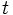$t$.

### Form of the differential equation

A (one-dimensional and degree one) second-order autonomous differential equation is a differential equation of the form: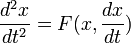$\frac{d^2x}{dt^2} = F(x, \frac{dx}{dt})$

### Solution method and formula

We set a variable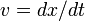$v = dx/dt$ Then, we can rewrite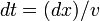$dt = (dx)/v$. In particular,$d^2x/dt^2 = (d/dt)(dx/dt) = dv/dt = dv/((dx)/v) = vdv/dx$. Plug this in:$v \frac{dv}{dx} = F(x, v)$

Solve this to obtain the general solution for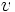$v$ in terms of$x$. Plug this expression in:$\frac{dx}{dt} = v(x)$

and solve this first-order differential equation. Note that if$v$ is not expressible as an explicit function of$x$, but we instead have a relational solution$R(x,v) = 0$, then solve the first-order differential equation: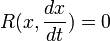$R(x, \frac{dx}{dt}) = 0$

## Particular cases

### Case where the function on the right depends only on$x$ and not on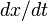$dx/dt$

Consider a situation of the form: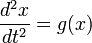$\frac{d^2x}{dt^2} = g(x)$

We do the same substitution$v = dx/dt$ and obtain: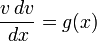$\frac{v \, dv}{ \, dx} = g(x)$

This is now a separable differential equation relating$x$ and$v$. Integrate and obtain:$\int v \, dv = \int g(x) \, dx$

We thus get: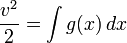$\frac{v^2}{2} = \int g(x) \, dx$

In particular, if$G$ is an antiderivative for$g$, then we get: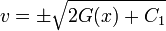$v = \pm \sqrt{2G(x) + C_1}$

where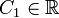$C_1 \in \R$ is a parameter. Each choice of$C_1$ gives a different solution.

Plug this back in and get:$\frac{dx}{dt} = \pm \sqrt{2G(x) + C_1}$

(The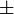$\pm$ indicates that there are in fact two differential equations and we need to take the union of their solution sets).

This is a first-order autonomous differential equation, and in particular a separable differential equation. Rearrange and get: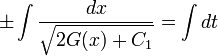$\pm \int \frac{dx}{\sqrt{2G(x) + C_1}} = \int dt$

An additional constant,$C_2$, arises from this indefinite integration. The upshot is that the general solution relates$x$ to$t$ and has two parameters$C_1,C_2$, as we might expect from the degree of the equation.

### Case where the function on the right is multiplicatively separable

Consider a situation of the form: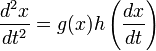$\frac{d^2x}{dt^2} = g(x)h\left(\frac{dx}{dt}\right)$

We do the same substitution$v = dx/dt$ and obtain: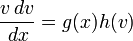$\frac{v \, dv}{ \, dx} = g(x)h(v)$

This is a separable differential equation and we can rearrange it to obtain: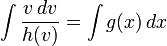$\int \frac{v \, dv}{h(v)} = \int g(x) \, dx$

We now perform the integration both sides. Suppose$H(v) = \int \frac{v \, dv}{h(v)}$ and$G(x) =\int g(x) \, dx$. We get: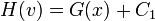$H(v) = G(x) + C_1$

If$H$ can locally be inverted, we can write$v$ as an explicit function of$x$ We now plug this into the original differential equation and get: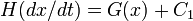$H(dx/dt) = G(x) + C_1$

This is now a first-order differential equation. If$H$ can locally be inverted, we can write$dx/dt$ as an explicit function of$x$ (locally) and then solve the resultant separable differential equation. Otherwise, there may be some other method available.

## Example

### Separable example

Consider the following differential equation:$\frac{d^2x}{dt^2} = 2x\frac{dx}{dt}$

We describe how to solve this using the technique discussed here. First, set$v = dx/dt$. We get: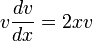$v \frac{dv}{dx} = 2xv$

Thus, we get:$v = 0 \qquad \mbox{ or } \qquad \frac{dv}{dx} = 2x$

The case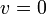$v = 0$ solves to gives$x = C_0, C_0 \in \R$

The case: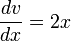$\frac{dv}{dx} = 2x$

solves to give: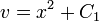$v = x^2 + C_1$

Now, we plug back the original substitution to get:$\frac{dx}{dt} = x^2 + C_1$

We now make cases based on the sign of$C_1$.

#### Case$C_1 = 0$

In this case, the differential equation becomes: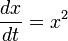$\frac{dx}{dt} = x^2$

This has a stationary solution$x = 0$ (which, incidentally, is already included in the prior solution family of all constant solutions) and the general solution can be obtained as:$\int \frac{dx}{x^2} = \int dt$

This gives:$\frac{-1}{x} = t + C_2$

This solves to give:$x = \frac{-1}{t + C_2}$

To verify that this is a solution, we compute, using the chain rule for differentiation and differentiation rule for power functions: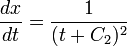$\frac{dx}{dt} = \frac{1}{(t + C_2)^2}$

and: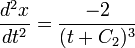$\frac{d^2x}{dt^2} = \frac{-2}{(t + C_2)^3}$

We can now plug these into the original differential equation and verify.

#### Case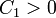$C_1 > 0$

Let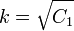$k = \sqrt{C_1}$. The differential equation becomes: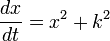$\frac{dx}{dt} = x^2 + k^2$

There are no stationary solutions. The general solution is given by: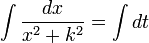$\int \frac{dx}{x^2 + k^2} = \int dt$

This solves to give:$\frac{1}{k} \arctan\left(\frac{x}{k}\right) = t + C_2$

Rearranging, we get: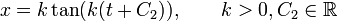$x = k \tan(k(t + C_2)), \qquad k > 0, C_2 \in \R$

Note that a priori, applying tan to both sides causes us to lose the information that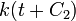$k(t + C_2)$ is in the range of$\arctan$. However, this constraint is "artificial" anyway and can be got rid of by readjusting the value of$C_2$. Note that arc tangent constraints are artificial, but arc sine constraints are genuine, because the sine function repeats itself within a period but the tangent function does not.

To verify that this is a solution, we can compute: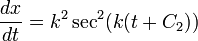$\frac{dx}{dt} = k^2 \sec^2(k(t + C_2))$$\frac{d^2x}{dt^2} = 2k^3 \sec^2(k(t + C_2)) \tan(k(t + C_2))$

We can now plug in and verify the original differential equation.

#### Case$C_1 < 0$

Let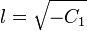$l = \sqrt{-C_1}$. The differential equation becomes: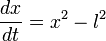$\frac{dx}{dt} = x^2 - l^2$

There are stationary solutions corresponding to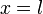$x = l$ and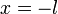$x = -l$. However, all stationary solutions are already included in another solution family, so we can ignore these. We continue solving: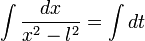$\int \frac{dx}{x^2 - l^2} = \int dt$

We get: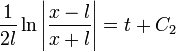$\frac{1}{2l} \ln \left|\frac{x - l}{x + l}\right| = t + C_2$

This rearranges to gives: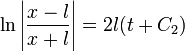$\ln \left|\frac{x - l}{x + l}\right| = 2l(t + C_2)$

Exponentiate both sides to get: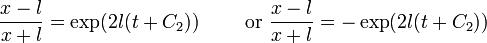$\frac{x - l}{x + l} = \exp(2l(t + C_2)) \qquad \mbox{ or } \frac{x - l}{x + l} = -\exp(2l(t + C_2))$

The solutions are respectively:$x = l\left(\frac{1 + \exp(2l(t + C_2))}{1 - \exp(2l(t + C_2))}\right) \qquad \mbox{ or } x = l\left(\frac{1 - \exp(2l(t + C_2))}{1 + \exp(2l(t + C_2))}\right)$

#### All cases

The following are the solution families:

•$x = C_0, C_0 \in \R$ (this is a singular solution family)
•$x = \frac{-1}{t + C_2}, C_2 \in \R$
•$x = k \tan(k(t + C_2)), \qquad k > 0, C_2 \in \R$
•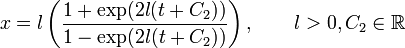$x = l\left(\frac{1 + \exp(2l(t + C_2))}{1 - \exp(2l(t + C_2))}\right), \qquad l > 0, C_2 \in \R$
•$x = l\left(\frac{1 - \exp(2l(t + C_2))}{1 + \exp(2l(t + C_2))}\right), \qquad l > 0, C_2 \in \R$